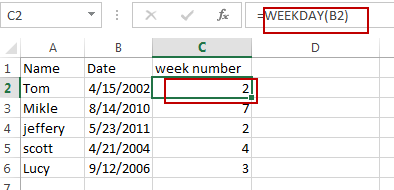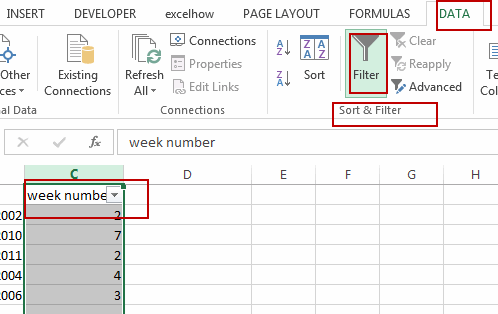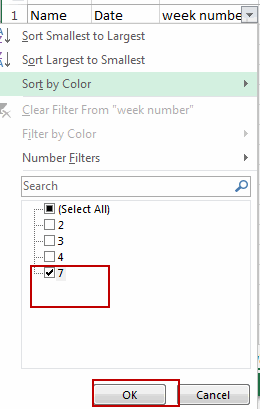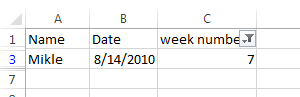# Filter Out Weekends and Weekday

This post will guide you how to filter out weekend or weekday from a list of data in Excel. How do I filter out the data in all Weekends in Excel. How to filter out all data in all weekdays in Excel.

## Filter Out data in Weekdays and Weekends

If you want to filter out the data that belong to all the weekends or weekdays in Excel, how to achieve it.  You can use a formula based on the WEEKDAY function to return a number 1-7 for each day of the week.  The number 1 indicates that it is Sunday, and the number 7 indicates that it is Saturday.  Then you can use the Filter feature to filter out the data based on the week number. Just do the following steps:

#1 Type the following formula in a cell adjacent to the date column.

`=WEEKDAY(B2)`

#2 press Enter key in your keyboard, and then drag the AutoFill Handle to other cells to apply this formula.#3 keeping the week numbers are selected, and go to DATA tab, click Filter command under Sort & Filter group.#4 click Arrow button in week number filed, checked 1 and 7 to filter out all weekends. Or checked 2-6 to filter out all weekdays. Click OK button.#5 let’s see the result.### Related Functions

• Excel WEEKDAY function
The Excel WEEKDAY function returns a integer value representing the day fo the week for a given Excel date and the value is range from 1 to 7.The syntax of the WEEKDAY function is as below:=WEEKDAY (serial_number,[return_type])…
Related Posts

Calculate Cumulative Loan Principal Payments in Excel

How do you Calculate Cumulative Loan Principal Payments in Excel? There are a few different ways to calculate this information, but one way has been proven to be accurate more often than others. One option is to use the CUMPRINC ...

Trap Error or Replace Error by Specific Value with IFERROR function

We often use Excel formulas in our work life, and we may encounter this situation that the formula throws an error and finally an error like #DIV/0! Is displayed in the cell. In today’s tutorial, we will introduce you the ...

Calculate Compound Interest in Excel

This article will show you how to calculate compound interest in Excel. It will help you calculate the interest you will accrue on a given amount of money. There are several ways to calculate compound interest. One of the simplest ...

Count Attendance and Absence with COUNTIF function

In our campus life and work life, we usually record everyone's attendance. Today we will introduce you the application of Excel COUNTIF function to count the attendance. Using a week as an example, we use the following example to show ...

Find the Closest Data to the Data Provided in Excel

In our daily work, we may encounter such an issue that to find the closest value to a certain value. In fact, Excel internal functions can help us solve this problem. In today’s article, we will show you how to ...

Sidebar